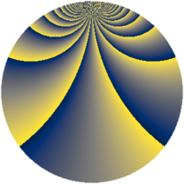# Properties

 Label 729.2.kLevel $729$ Weight $2$ Character orbit 729.k Rep. character $\chi_{729}(4,\cdot)$ Character field $\Q(\zeta_{243})$ Dimension $12960$ Newform subspaces $1$ Sturm bound $162$ Trace bound $0$

# Related objects

## Defining parameters

 Level: $$N$$ $$=$$ $$729 = 3^{6}$$ Weight: $$k$$ $$=$$ $$2$$ Character orbit: $$[\chi]$$ $$=$$ 729.k (of order $$243$$ and degree $$162$$) Character conductor: $$\operatorname{cond}(\chi)$$ $$=$$ $$729$$ Character field: $$\Q(\zeta_{243})$$ Newform subspaces: $$1$$ Sturm bound: $$162$$ Trace bound: $$0$$

## Dimensions

The following table gives the dimensions of various subspaces of $$M_{2}(729, [\chi])$$.

Total New Old
Modular forms 13284 13284 0
Cusp forms 12960 12960 0
Eisenstein series 324 324 0

## Trace form

 $$12960 q - 162 q^{2} - 162 q^{3} - 162 q^{4} - 162 q^{5} - 162 q^{6} - 162 q^{7} - 162 q^{8} - 162 q^{9} + O(q^{10})$$ $$12960 q - 162 q^{2} - 162 q^{3} - 162 q^{4} - 162 q^{5} - 162 q^{6} - 162 q^{7} - 162 q^{8} - 162 q^{9} - 162 q^{10} - 162 q^{11} - 162 q^{12} - 162 q^{13} - 162 q^{14} - 162 q^{15} - 162 q^{16} - 162 q^{17} - 162 q^{18} - 162 q^{19} - 162 q^{20} - 162 q^{21} - 162 q^{22} - 162 q^{23} - 162 q^{24} - 162 q^{25} - 162 q^{26} - 162 q^{27} - 162 q^{28} - 162 q^{29} - 162 q^{30} - 162 q^{31} - 162 q^{32} - 162 q^{33} - 162 q^{34} - 162 q^{35} - 162 q^{36} - 162 q^{37} - 162 q^{38} - 162 q^{39} - 162 q^{40} - 162 q^{41} - 162 q^{42} - 162 q^{43} - 162 q^{44} - 162 q^{45} - 162 q^{46} - 162 q^{47} - 162 q^{48} - 162 q^{49} - 162 q^{50} - 162 q^{51} - 162 q^{52} - 162 q^{53} - 162 q^{54} - 162 q^{55} - 162 q^{56} - 162 q^{57} - 162 q^{58} - 162 q^{59} - 162 q^{60} - 162 q^{61} - 162 q^{62} - 162 q^{63} - 162 q^{64} - 162 q^{65} - 162 q^{66} - 162 q^{67} - 162 q^{68} - 162 q^{69} - 162 q^{70} - 162 q^{71} - 162 q^{72} - 162 q^{73} - 162 q^{74} - 162 q^{75} - 162 q^{76} - 162 q^{77} - 162 q^{78} - 162 q^{79} - 162 q^{80} - 162 q^{81} - 162 q^{82} - 162 q^{83} - 162 q^{84} - 162 q^{85} - 162 q^{86} - 162 q^{87} - 162 q^{88} - 162 q^{89} - 162 q^{90} - 162 q^{91} - 162 q^{92} - 162 q^{93} - 162 q^{94} - 162 q^{95} - 162 q^{96} - 162 q^{97} - 162 q^{98} - 162 q^{99} + O(q^{100})$$

## Decomposition of $$S_{2}^{\mathrm{new}}(729, [\chi])$$ into newform subspaces

Label Dim $A$ Field CM Traces $q$-expansion
$a_{2}$ $a_{3}$ $a_{5}$ $a_{7}$
729.2.k.a $12960$ $5.821$ None $$-162$$ $$-162$$ $$-162$$ $$-162$$